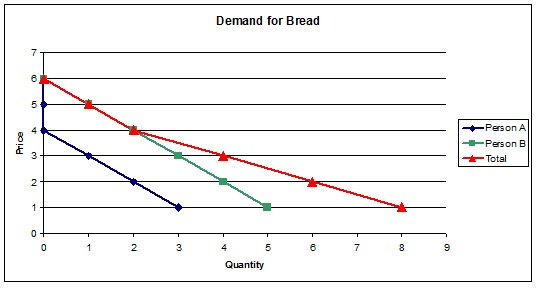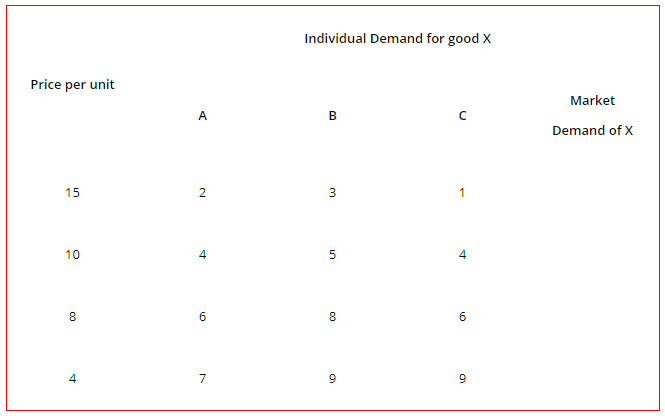# Market Demand: Graphical Representation, Concepts, Examples

The compilation of these Consumer Behaviour Notes makes students exam preparation simpler and organised.

## Market Demand

The consumption of a good or service at different price levels can be depicted for a single consumer using the demand curve. But how do we depict the total consumption for that commodity from all consumers in the market? Read this lesson on Market demand that explains how to calculate and even graphically represent total market demand.

### Consumer’s Demand

In economics, ‘demand’ refers to the quantity of a good or service that consumers are willing and able to purchase at a given price. Different from what consumers desire to purchase, demand explains what they are actually able to purchase. Not all desires can be met for the reason that goods are guided by prices in the market. At higher prices, consumers would want to buy less of a good and the reverse is true for lower prices. This relation is the famous law of demand.

Another reason that desires are not the same as actual consumer decisions is that consumers are constrained by their budget. The money income of a consumer will limit his ability to purchase a commodity. Thus, demand expresses both willingness and ability to consume. Willingness is depicted by consumer preferences and ability is depicted by constraints such as money income and prices (budget constraint).

### Market Demand

Individual demand is the demand of a single consumer for a good or service at a given price, with other factors as money income, tastes, and preferences, prices of other goods constant. It can be graphically depicted by a downward sloping demand curve for a single consumer. The curve represents different price-quantity combinations available to a consumer to consume.

Market demand refers to the demand of all consumers of a good or service at a given price, with other factors as money income, tastes, and preferences, prices of other goods constant. It is called ‘market’ demand because it depicts the market situation for a good or service. It can be graphically obtained by aggregating the individuals’ consumer demand for a commodity. In simple words, the horizontal summation of all individual demand curves for a good or service gives you the market demand curve.

Market Demand Curve
The pre-requisite for drawing a market demand curve is that all individual demand curves must be known. It is simple to then draw the market demand curve from the market demand schedule. By summing the individual demands at different prices, we can get different price-quantity combinations for the market demand curve.

The law of demand holds for the market demand curve also i.e. it slopes downwards. If the market size is large, it is possible to take a small group of representative consumers and multiply their average quantities by the total number of consumers in the market to obtain the market demand for that good.

Graphical Representation of Market Demand CurveIn the graph above, we assume that there are only two consumers in a market for purposes of understanding. Let them be Person A and Person B. The individual demand curves for A and B can be drawn from their choice of consumption at different prices. The demand curves are drawn in blue and green for A and B respectively. Note that A demands nothing at prices above Rs. 3 so his demand curve coincides with the vertical axis. B demands some quantity at these prices but nothing at Rs. 6. At prices below and equal to Rs. 3, both consumers demand the good (here, bread).

The red curve depicts the market demand, obtained by horizontally summing A and B’s consumption preferences at different prices. For example, A and B demand nothing at Rs. 6 so the demand curve starts from the vertical intercept where price equals 6. At Rs. 5, A demands nothing but B demands 1 unit of bread, so the market demand becomes the sum of both, which is one unit only. Even at Rs.4, B demands something but A does not, so till Rs. 4, the market demand curve coincides with the demand curve of B.

At Rs. 3, A demands 1 unit and B demands 3 units, so the market demand becomes 4 units (by summing). This can be read from the curve at Rs. 3. Similarly, the graph can be read for other price levels. Thus, you can understand how the market demand curve is derived. If the individual demand schedules for consumers are given, you should now be able to sum quantities demanded at different prices and derive the market demand curve.

Example:

Question:
Complete the table below to determine market demandSolution:
We know that Market demand is the horizontal summation of individual demands of a commodity at different prices. Therefore, here, we sum across the rows to obtain market demand for good X at each price.We have horizontally summed over the quantities demanded by A, B, and C to obtain the required demand of the market.